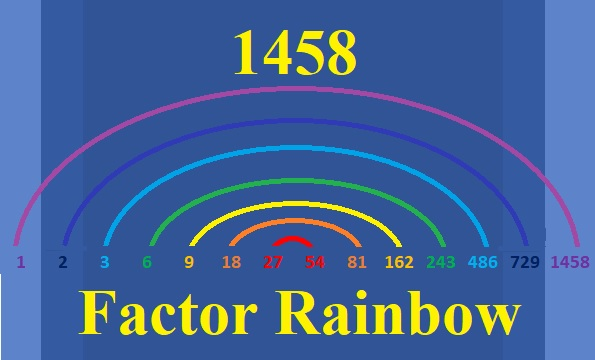# 1458 Tangrams Can Be A Pot of Gold

### A Tangram Puzzle

Tangrams are seven puzzle pieces that can form a square but can also be made into many different people, places, and things. A lot of stress is going on in the world right now, but since tomorrow is Saint Patrick’s Day, we can still find a little pot of gold at the end of the rainbow!

I made this pot of gold on Desmos using points and equations. If you cut it apart, will you be able to put it back together again?What other things can you make from those seven tangram shapes?

And what about that rainbow I mentioned? The number 1458 makes a lovely factor rainbow.

#### A Factor Rainbow for 1458:#### Factors of the number 1458:

• 1458 is a composite number.
• Prime factorization: 1458 = 2 × 3 × 3 × 3 × 3 × 3 × 3, which can be written 1458 = 2 × 3⁶.
• 1458 has at least one exponent greater than 1 in its prime factorization so √1458 can be simplified. Taking the factor pair from the factor pair table below with the largest square number factor, we get √1458 = (√729)(√2) = 27√2
• The exponents in the prime factorization are 1 and 6. Adding one to each exponent and multiplying we get (1 + 1)(6 + 1) = 2 × 7 = 14. Therefore 1458 has exactly 14 factors.
• The factors of 1458 are outlined with their factor pair partners in the graphic below.#### More Facts about the Number 1458:

2 is a prime factor of 1458 exactly one time, so there are NO ways that 1458 can be written as the difference of two squares.

2 and 3 are the only primes appearing in its prime factorization, so 1458 is NEVER the hypotenuse of a Pythagorean triple.

Nevertheless, since there are three different ways that 1458 = 2(a)(b), where a > b, there are three ways that 1458 is a leg in a Pythagorean triple:
1458-531440-531442, calculated from 2(729)(1), 729² – 1², 729² + 1²
1458-59040-59058, calculated from 2(243)(3), 243² – 3², 243² + 3²
1458-6480-6642, calculated from 2(81)(9), 81² – 9², 81² + 9²

Why can’t we get a Pythagorean triple from 1458 = 2(27)(27)? I’m sure you can figure out that one yourself.

This site uses Akismet to reduce spam. Learn how your comment data is processed.